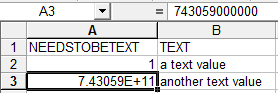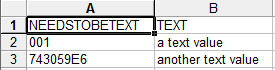# Forcing values to be inserted into spreadsheets as text

13 April 2011

An enhancement that I meant to include in my Simplicity of CF9 posts is `<cfspreadsheet>` and the related script functions.

It's not quite as simple as it might be though. Today I wanted to export a recordset as a spreadsheet, but certain string values were being treated as numbers when added to the sheet. Here's a basic example:

``````<cfscript>
// Create a 2 column, 2 row query. The first column contains numbers or possible numbers we want formatted as text in our spreadsheet
q = QueryNew( "" );
QueryAddColumn( q,"Text","VarChar",[ "a text value","another text value" ] );
// Use the query column names as column headers in our sheet
filename = "test.xls";
</cfscript>
<cfcontent type="application/msexcel" variable="#sheetAsBinary#" reset="true">``````

And here's the result:As you can see both values in the first column have been interpreted as numbers rather than strings.

I scoured the CF Docs and further afield, but it seems you cannot pre-format a column or row to stop the auto-interpretation when adding data. You can apply formatting afterwards, but by then it's too late: the wrong values have been added.

However, it seems that CF9.0.1 does allow you to format cells as text and then use the `SpreadsheetSetCellValue()` function which will respect the given format.

So I set about trying to use this feature and came up with a 3-step process which seems to do the job:

1. Populate the spreadsheet, ignoring the incorrectly interpreted number values.
2. Format the column I want to contain text values.
3. Replace the incorrect value in each row of that column with the correct value, which will now be treated as text.

Wrapped up as a function it looks like this:

``````function spreadsheetAddRowsForceTextFormat
(
,required query data
,required array columnNumbersToFormatAsText
)
{
{
// Get a list of the query column names in the order they appear, as opposed to the alphabetical columnList
}
// Add the data: the numbers will be inserted as numeric for now
for( var columnNumberToFormat in arguments.columnNumbersToFormatAsText )
{
// Now we format the column as text
// Having formatted the column, add the column from our query again so the values correct
while( arguments.data.next() )
{
local.rownumber = arguments.data.currentrow;
local.rownumber++; // start one row below to allow for header
// Get the value of column at the current row in the loop
local.value = arguments.data[ local.columns[ columnNumberToFormat ] ][ arguments.data.currentrow ];
// replace the previously added numeric value which will now be treated as text
}
}
}``````

You specify the columns you want as text using an array, which allows several columns at once. There's also an option to exclude the header row if required.

IMPORTANT UPDATE 14 April 2011: I originally used the `columnList` property to get the query columns, but this is always alphabetical and so may not match the order of columns in the query. Instead I'm now using `getMetaData().getColumnLabels()` which preserves the original order.

My test code now looks like this:

``````<cfscript>
// Create a 2 column, 2 row query. The first column contains numbers or possible numbers we want formatted as text in our spreadsheet
q = QueryNew( "" );
QueryAddColumn( q,"Text","VarChar",[ "a text value","another text value" ] );
(
,data=q
,columnNumbersToFormatAsText=[ 1 ]
);
filename = "test.xls";
</cfscript>
<cfcontent type="application/msexcel" variable="#sheetAsBinary#" reset="true">``````The column is now correctly showing the original values as text strings.

## Update 25 November 2013

Since the new faster spreadsheet population function handles the header row, you only need to tell the formatting function whether or not the sheet includes a header.

``````function spreadsheetForceTextFormat
(
,required query data
,required array columnNumbersToFormatAsText
){
var rownumber=0;
var value="";
for( var columnNumberToFormat in columnNumbersToFormatAsText )
{
while( data.next() )
{
rownumber	=	data.currentrow;
rownumber++; // start one row below to allow for header
// Get the value of column at the current row in the loop
value	=	data[ columns[ columnNumberToFormat ] ][ data.currentrow ];
// replace the previously added numeric value which will now be treated as text
}
}
}``````

The calling code now looks like this.

``````<cfscript>
// Create a 2 column, 2 row query. The first column contains numbers or possible numbers we want formatted as text in our spreadsheet
q = QueryNew( "" );
QueryAddColumn( q,"Text","VarChar",[ "a text value","another text value" ] );
(
,data=q
,columnNumbersToFormatAsText=[ 1 ]
);